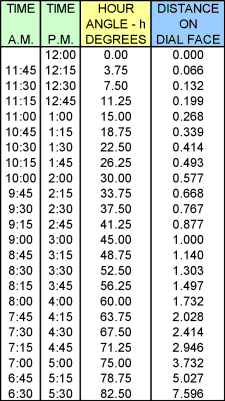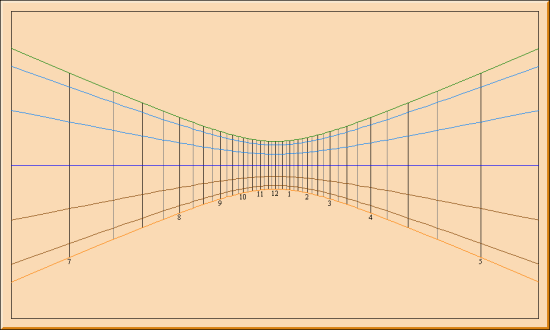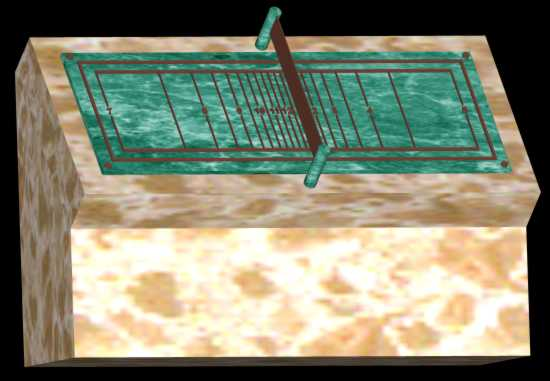The Sundial Primer created by Carl SabanskiThe Sundial Primer Index
 Polar Sundial Polar Sundial: is a sundial in which the dial plate is set along the East-West direction and inclines so that it is parallel with the polar axis. The standard polar pointing gnomon is thus also parallel to the dial plate. Latitude: is the angular position of a place north or south of the equator. Positive values in the Northern hemisphere, negative in the Southern hemisphere. Style Height / Style Angle (SH): of a polar style is the angle that the style makes with the sub-style line. Sub-Style (line): the line lying in the dial plane which is perpendicularly below (or behind for a vertical dial) the style. Hour Line: the line on a dial plate indicating the shadow position at a particular time (includes fractional as well as whole hours). Hour Angle (h, HA): the angle corresponding to the sun's position around its daily (apparent) orbit. Measured westward from local noon, it increases at a rate of 15° per hour. Thus 3 pm (Local Apparent Time) is 45° and 9 am is -45° The polar sundial is universal and can be used at any latitude. The hour lines are parallel to each other and are symmetrical with respect to the noon line. The gnomon is positioned on the noon hour line with its style parallel to the dial plate. The dial plate lies parallel to the Earth's axis, i.e. it is tilted to an angle equal to the latitude where it is located. As the distance to the 6 am and 6 pm hour lines approach infinity, the time that can be indicated by the dial is limited to the practical width of the dial plate. The distance between the hour lines and the sub-style can be calculated as follows. In this case the sub-style is the noon hour-line. If the gnomon has an appreciable thickness, then a noon gap would appear and two sub-styles would exist. By choosing a gnomon height GH:X =  GH * tan (h) where h is the hour angle, in degrees, given by:h = (T24 - 12) * 15°and T24 is the time in 24-hour clock notation (hours after midnight) in decimal hours.Table 1 list the distance to the hour lines for 15-minute intervals assuming a gnomon height of 1. By selecting a gnomon height you can easily determine the distance to each hour line by using the multipliers. The number of hours displayed can be determined by selecting the dial width. Click here to download a spreadsheet that will perform these calculations for you.Table 1: Polar Sundial Hour Line DistancesNote that the distance to the 9 am and 3 pm hour lines is equal to the height of the gnomon. As an example, selecting a gnomon height of 2.5 inches will result in a dial width of 37.98 inches for a polar sundial dial that indicates time between 6:30 am and 5:30 pm.Figure 1 illustrates the layout of the hour lines for a polar dial and how it must be installed to function correctly at any latitude.Figure 1: Polar SundialRather than using a plate for the gnomon, a vertical rod can be used to create a nodus. It is then possible to show the declination lines on the dial plate. Figure 2 illustrates such an example. The height of the nodus will affect the shadow path throughout the year and the number of hours that can be displayed on a dial plate of a given size.Figure 2: Polar Sundial with Declination Lines (SHADOWS)For an image complete with shadow click here.